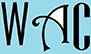## Algebra Question for Title 1 Schools: What is the Value Question?

12) Ehi pays \$1,100 in taxes for a van, what is the value of the van if the tax rate is 8.9%?

8.9% = 8.9/100 = 0.089

x = value of van

0.089x = 1100

you have to make “x” subject of formula by moving all the “x” to one side. When you go to the other side, addition becomes subtraction, multiplication becomes division, and vice versa.

x = 1100/0.089 (0.089 was multiplication at the other end)

x = \$12,359.55